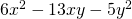Chapter 7: Factoring

# 7.4 Factoring Trinomials where a ≠ 1

Factoring trinomials where the leading term is not 1 is only slightly more difficult than when the leading coefficient is 1. The method used to factor the trinomial is unchanged.

Example 7.4.1

Factor the trinomial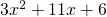.

Start by multiplying the coefficients from the first and the last terms. This is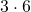, which yields 18.

The next task is to find all possible integers that multiply to 18 and their sums.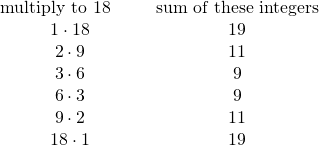Look for the pair of integers that multiplies to 18 and adds to 11, so that it matches the equation that you started with.

For this example, the pair is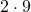, which adds to 11.

Now take the original trinomialand break the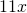into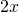and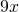.

Rewrite the original trinomial as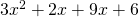.

Now, split this into two binomials as done in the previous section and factor.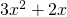yields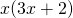and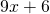yields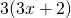.becomes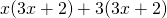.factors to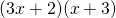.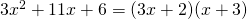The master product method works for any integer breakup of the polynomial. Slightly more complicated are questions that involve two different variables in the original polynomial.

Example 7.4.2

Factor the trinomial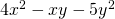.

Start by multiplying the coefficients from the first and the last terms. This is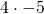, which yields −20.

The next task is to find all possible integers that multiply to −20 and their sums.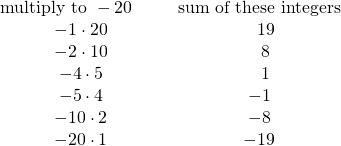Look for the pair of integers that multiplies to −20 and adds to −11, so that it matches the equation that you started with.

For this example, the pair is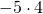, which adds to −1.

Now take the original trinomialand break the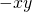into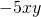and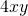.

Rewrite the original trinomial as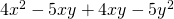.

Now, split this into two binomials as done in the previous section and factor.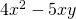yields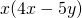and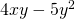yields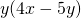.becomes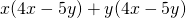.factors to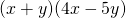.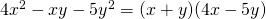There are a number of variations potentially encountered when factoring trinomials. For instance, the original terms might be mixed up. There could be something like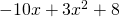that is not in descending powers of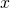. This requires reordering in descending powers before beginning to factor.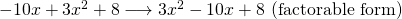It might also be necessary to factor out a common factor before starting. The polynomial above can be written as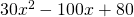, in which a common factor of 10 should be factored out prior to factoring.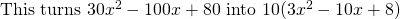.

There are also slight variations on the common factored binomial that can be illustrated by factoring the trinomial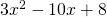.

Example 7.4.3

Factor the trinomial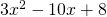.

Start by multiplying the coefficients from the first and the last terms. This is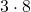, which yields 24.

The next task is to find all possible integers that multiply to 24 and their sums (knowing that the middle coefficient must be negative).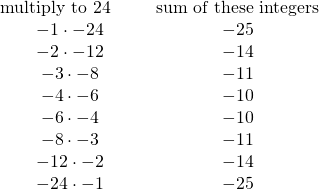Look for the pair of integers that multiplies to 24 and adds to −10, so that it matches the equation that you started with.

For this example, the pair is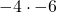, which adds to −10.

Now take the original trinomialand break the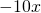into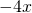and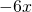.

Rewrite the original trinomial as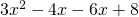.

Now, split this into two binomials as done in the previous section and factor.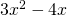yields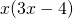, but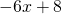yields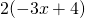.andare a close match, but their signs are different.

The way to deal with this is to factor out a negative, specifically, −2 instead of 2.can be factored two ways:and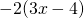.

Choose the second factoring, so the common factor matches.becomes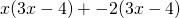.factors to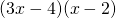.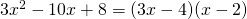Example 7.4.4

Factor the following trinomials, which are both variations of the trinomial seen before in 7.4.3:

1.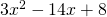The pair of numbers that can be used to break it up is −2 and −12.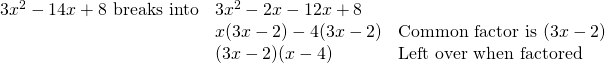2.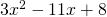The pair of numbers that can be used to break it up is −3 and −8.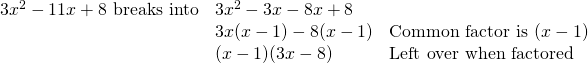# Questions

Factor each of the following trinomials.

1.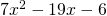2.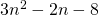3.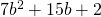4.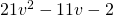5.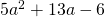6.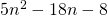7.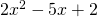8.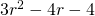9.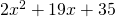10.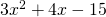11.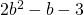12.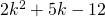13.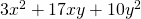14.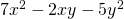15.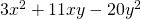16.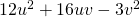17.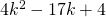18.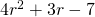19.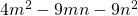20.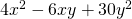21.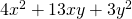22.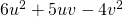23.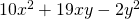24.